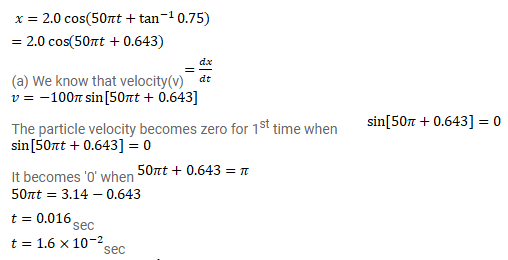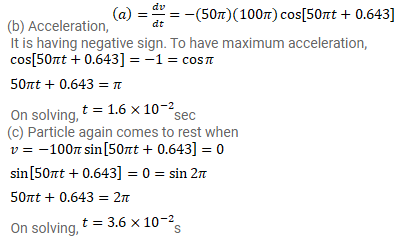# Consider a particle moving in simple harmonic motion according to the equation.

Question:

Consider a particle moving in simple harmonic motion according to the equation.

$x=2.0 \cos \left(50 \pi t+\tan ^{-1} 0.75\right)$

where $x$ is in centimeter and $t$ in second. The motion is started at $t=0$.

(a) When does the particle come to rest for the first time?

(b) When does the acceleration have its maximum magnitude for the first time?

(c) When does the particle come to rest for the second time?

Solution: